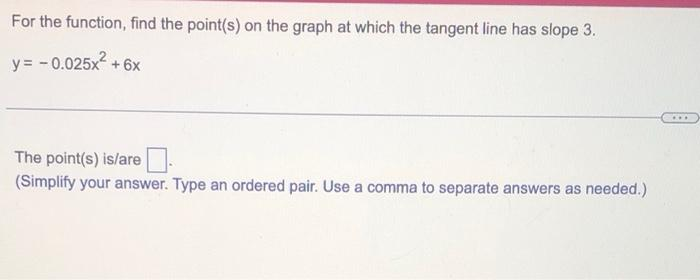Home / Expert Answers / Calculus / for-the-function-find-the-point-s-on-the-graph-at-which-the-tangent-line-has-slope-3-y-0-02-pa167

# (Solved): For the function, find the point(s) on the graph at which the tangent line has slope 3 . \[ y=-0.02 ...For the function, find the point(s) on the graph at which the tangent line has slope 3 . \[ y=-0.025 x^{2}+6 x \] The point(s) is/are (Simplify your answer. Type an ordered pair. Use a comma to separate answers as needed.)

We have an Answer from Expert